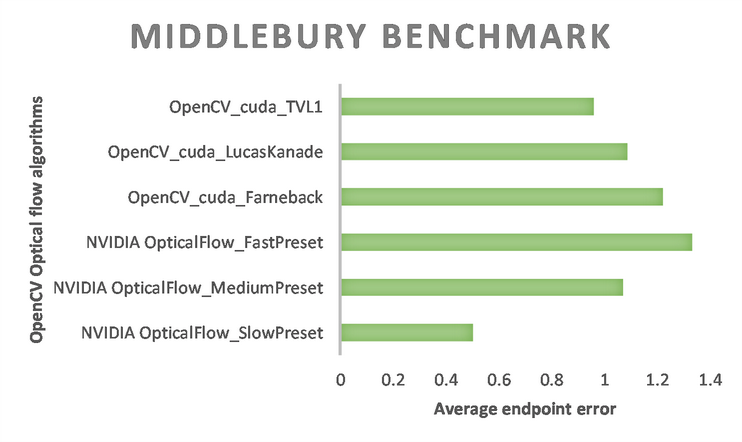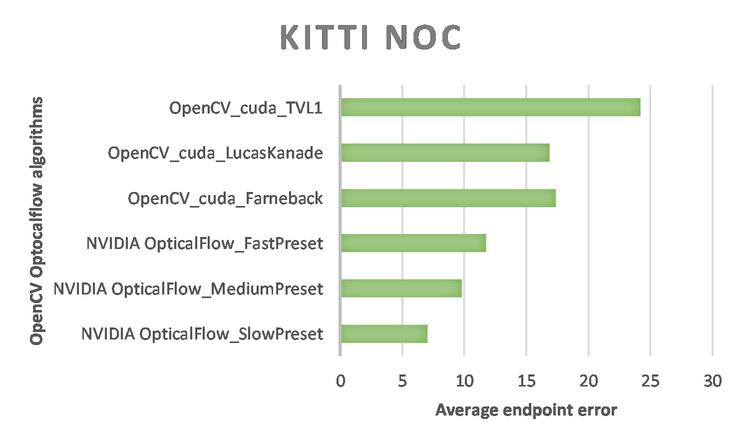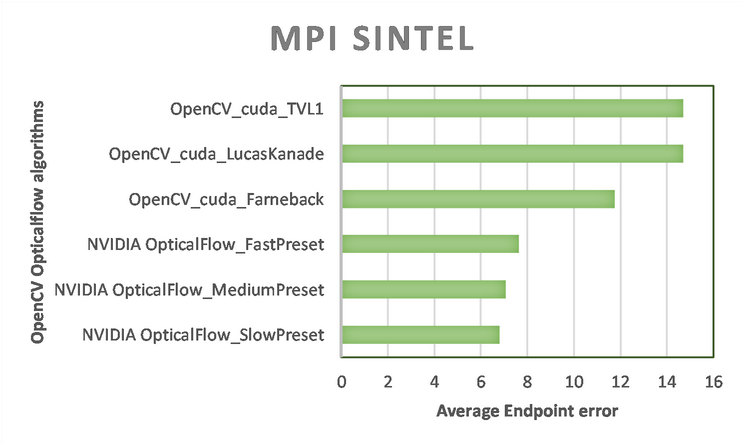# Accelerate OpenCV: Optical Flow Algorithms with NVIDIA Turing GPUsOpenCV is a popular open-source computer vision and machine learning software library with many computer vision algorithms including identifying objects, identifying actions, and tracking movements. The tracking algorithms use optical flow to compute motion vectors that represent the relative motion of pixels (and hence objects) between images. Computation of optical flow vectors is a computationally expensive task. However, OpenCV 4.1.1 introduces the ability to use hardware acceleration on NVIDIA Turing GPUs to dramatically accelerate optical flow calculation.

NVIDIA Turing GPUs include dedicated hardware for computing optical flow (OF). This dedicated hardware uses sophisticated algorithms to yield highly accurate flow vectors, which are robust to frame-to-frame intensity variations, and track true object motion. Computation is significantly faster than other methods at comparable accuracy.

The new NVIDIA hardware accelerated OpenCV interface is similar to that of other optical flow algorithms in OpenCV so developers can easily port and accelerate their existing optical flow based applications with minimal code changes. More details about the OpenCV integration can be found here.

## Optical Flow `calc` in OpenCV

OpenCV supports a number of optical flow algorithms. The pyramidal version of Lucas-Kanade method (`SparsePyrLKOpticalFlow`) computes the optical flow vectors for a sparse feature set. OpenCV also contains a dense version of pyramidal Lucas-Kanade optical flow. Many of these algorithms have CUDA-accelerated versions; for example `BroxOpticalFlow`, `FarnebackOpticalFlow`, `DualTVL1OpticalFlow`.

In all cases these classes implement a `calc` function which takes two input images and returns the flow vector field between them.

## Hardware-Accelerated Optical Flow

The `NvidiaHWOpticalFlow` class implements NVIDIA hardware-accelerated optical flow into OpenCV. This class implements a calc function similar to other OpenCV OF algorithms. The function takes two images as input and returns dense optical flow vectors between the input images. Optical flow is calculated on a dedicated hardware unit in the GPU silicon which leaves the streaming multiprocessors (typically used by CUDA programs) free to perform other tasks. The optical flow hardware returns fine grained flow vectors with quarter-pixel accuracy. The default granularity is 4×4 pixel blocks but can be further refined using various upsampling algorithms. Helper functions are available in class `NvidiaHWOpticalFlow` to increase the flow vector granularity to 1×1 (per-pixel) or 2×2 block sizes.

In addition to supporting the basic OpenCV-OF functionality, `NvidiaHWOpticalFlow` also provides features for low-level control and performance tuning. Presets help developers fine-tune the balance between performance and quality. Developers can also enable or disable temporal hints so that the hardware uses previously generated flow vectors as internal hints to calculate optical flow for the current pair of frames. This is useful when computing flow vectors between successive video frames. Programmers can also set external hints, if available, to aid the computation of flow vectors. The optical flow library also outputs the confidence for each generated flow vector in the form of cost. The cost is inversely proportional to the confidence of the flow vectors and allows the user to make application-level decisions; e.g. accept/reject the vectors based on a confidence threshold.

The results of four different optical flow algorithms in OpenCV are demonstrated in the above video. The video compares the time it takes to calculate the optical flow vectors between successive frames and shows GPU utilization. NVIDIA hardware optical flow is extremely fast, computing vectors in 2 to 3ms per frame, and highly accurate while consuming very little GPU. Comparatively Farneback takes ~8ms per frame and returns lower accuracy flow vectors. Lucas-Kanade takes well over 20ms per frame and also returns lower accuracy flow vectors. TVL1 provides the highest accuracy optical flow vectors, but is computationally very expensive taking over 300ms per frame.

## Installing `NvidiaHWOpticalFlow`

The OpenCV implementation of NVIDIA hardware optical flow leverages the NVIDIA Optical Flow SDK which is a set of APIs and libraries to access the hardware on NVIDIA Turing GPUs. Developers desiring low-level control can use the APIs exposed in the SDK to achieve the highest possible performance.

The new OpenCV interface provides an OpenCV-framework-compliant wrapper around the NVIDIA Optical Flow SDK. The objective of providing such a wrapper is to facilitate easy integration and drop-in-compatibility with other optical flow algorithms available in OpenCV.

The OpenCV implementation of NVIDIA hardware optical flow is available in the `contrib` branch of OpenCV. Follow the steps in the links below to install OpenCV `contrib` build along with its Python setup. Note that NVIDIA Optical flow SDK is a prerequisite for these steps and is installed by default as a git submodule.

If you have questions regarding installing or using `NvidiaHWOpticalFlow` please post your question on the Video Codec and Optical Flow SDK forum.

## Docker Configuration

While launching the Docker it is essential to configure the NVIDIA container library component (libnvidia-container) to expose the libraries required for encode, decode and optical flow.

This can be done by adding the following command line option when launching the docker:

`“ -e NVIDIA_DRIVER_CAPABILITIES=compute,video,utility”`

## Python Bindings

Accessing NVIDIA optical flow via Python helps deep learning applications that require optical flow vectors between frames.

OpenCV Python is a wrapper class for the original C++ library so it can be used with Python. A Python interface for `NvidiaHWOpticalFlow` class is also available. The OpenCV array structures gets converted to NumPy arrays which makes it easier to integrate with other libraries that use NumPy.

Here is a sample code which calculates optical flow between the two images basketball1.png and basketball2.png – both images are a part of the OpenCV samples:

```import numpy as np
import cv2

nvof = cv2.cuda_NvidiaOpticalFlow_1_0.create(frame1.shape, frame1.shape, 5, False, False, False, 0)

flow = nvof.calc(frame1, frame2, None)

flowUpSampled = nvof.upSampler(flow, frame1.shape, frame1.shape, nvof.getGridSize(), None)

cv2.writeOpticalFlow('OpticalFlow.flo', flowUpSampled)

nvof.collectGarbage()```

## Accuracy Comparison

Average end-point error (EPE) for a set of optical flow vectors is defined as the average of the Euclidian distance between the true flow vector (i.e. ground truth) and the flow vector calculated by the algorithm being evaluated. Lower average EPE generally implies better quality of the flow vectors.

Figure 2, Figure 3 and Figure 4 below show the EPE obtained for the Middlebury, KITTI 2015 and MPI Sintel benchmark datasets using various optical flow algorithms available in OpenCV.Figure 2. Accuracy comparison of optical flow vectors generated by various OpenCV algorithms on Middlebury datasetFigure 3. Accuracy comparison of optical flow vectors generated by various OpenCV algorithms on KITTI 2015 benchmarkFigure 4. Accuracy comparison of optical flow vectors generated by various OpenCV algorithms on MPI Sintel dataset

## References

1. OpenCV library
2. NVIDIA Optical Flow SDK
3. NVIDIA Optical Flow Programming Guide (requires program membership)
4. Developer Blog: An Introduction to the NVIDIA Optical Flow SDK